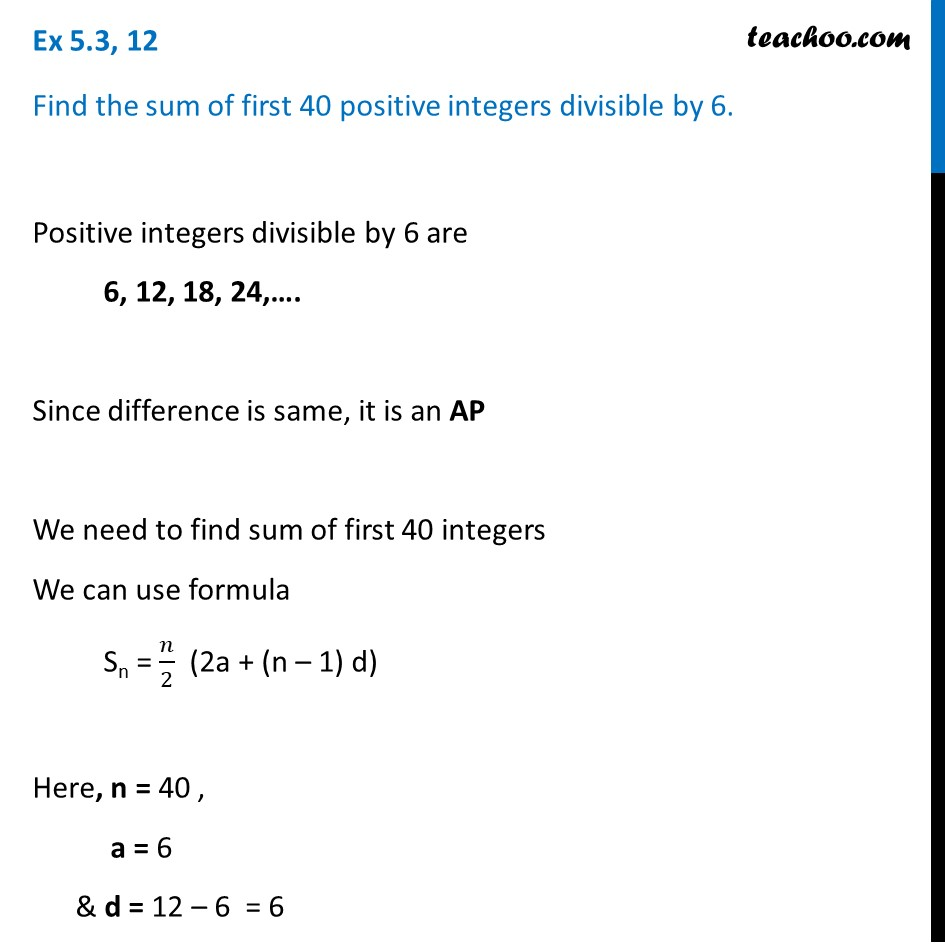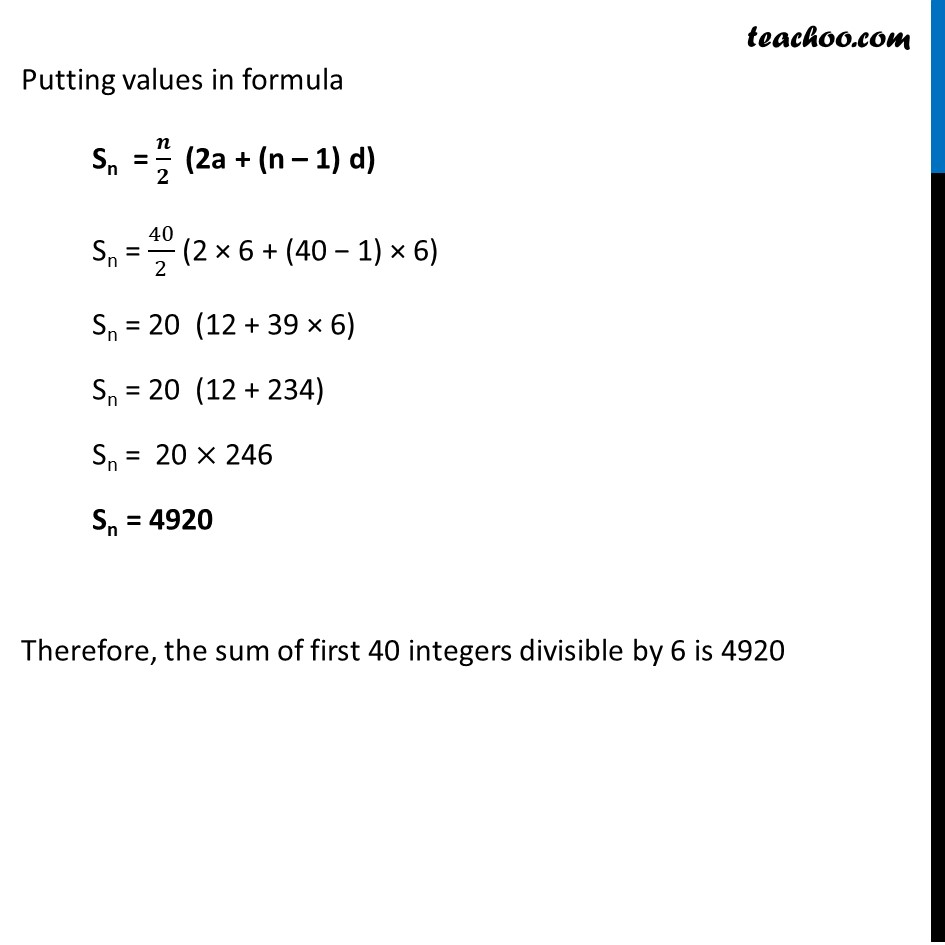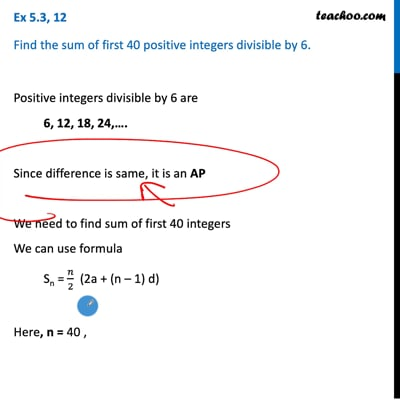Ex 5.3

Chapter 5 Class 10 Arithmetic Progressions
Serial order wiseThis video is only available for Teachoo black users

Solve all your doubts with Teachoo Black (new monthly pack available now!)

### Transcript

Ex 5.3, 12 Find the sum of first 40 positive integers divisible by 6. Positive integers divisible by 6 are 6, 12, 18, 24,…. Since difference is same, it is an AP We need to find sum of first 40 integers We can use formula Sn = 𝑛/2 (2a + (n – 1) d) Here, n = 40 , a = 6 & d = 12 – 6 = 6 Putting values in formula Sn = 𝒏/𝟐 (2a + (n – 1) d) Sn = 40/2 (2 × 6 + (40 − 1) × 6) Sn = 20 (12 + 39 × 6) Sn = 20 (12 + 234) Sn = 20 × 246 Sn = 4920 Therefore, the sum of first 40 integers divisible by 6 is 4920